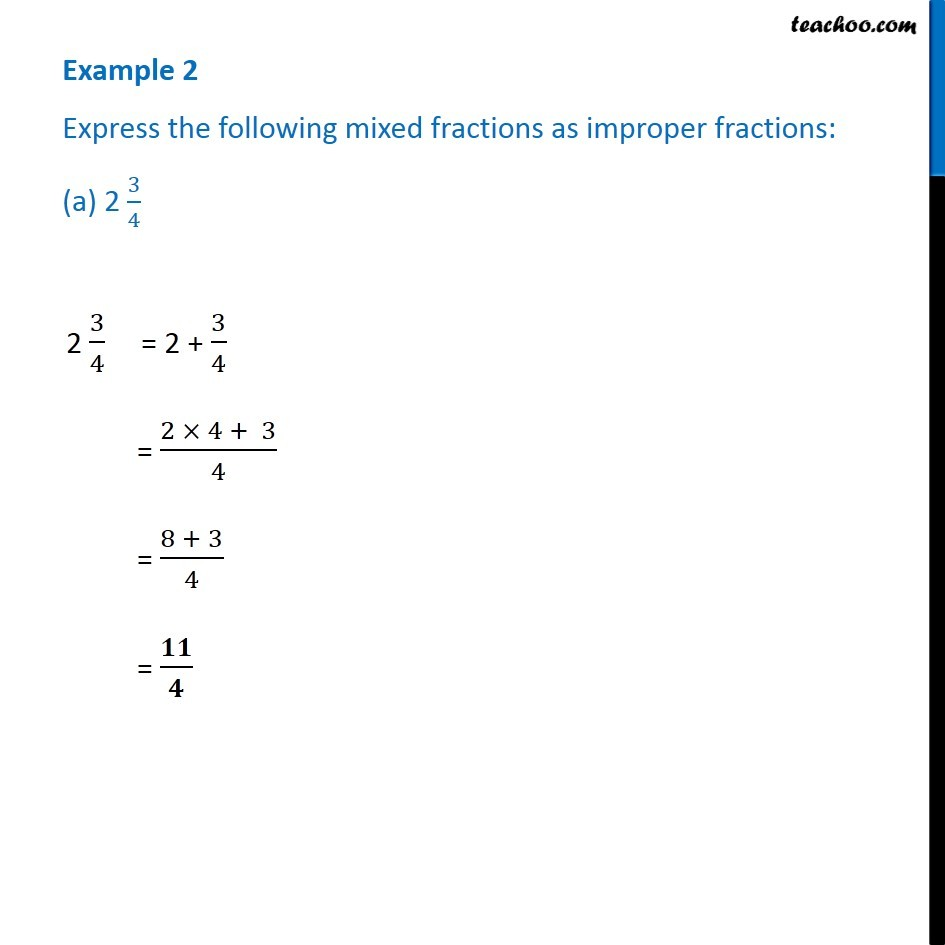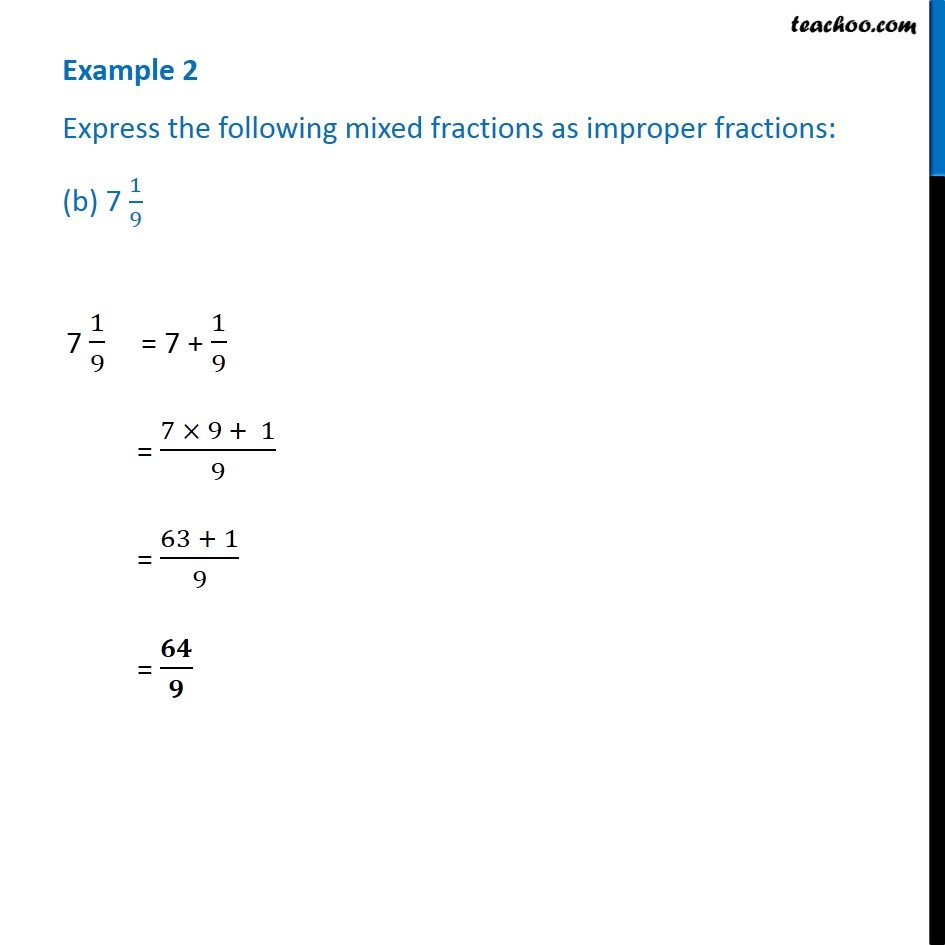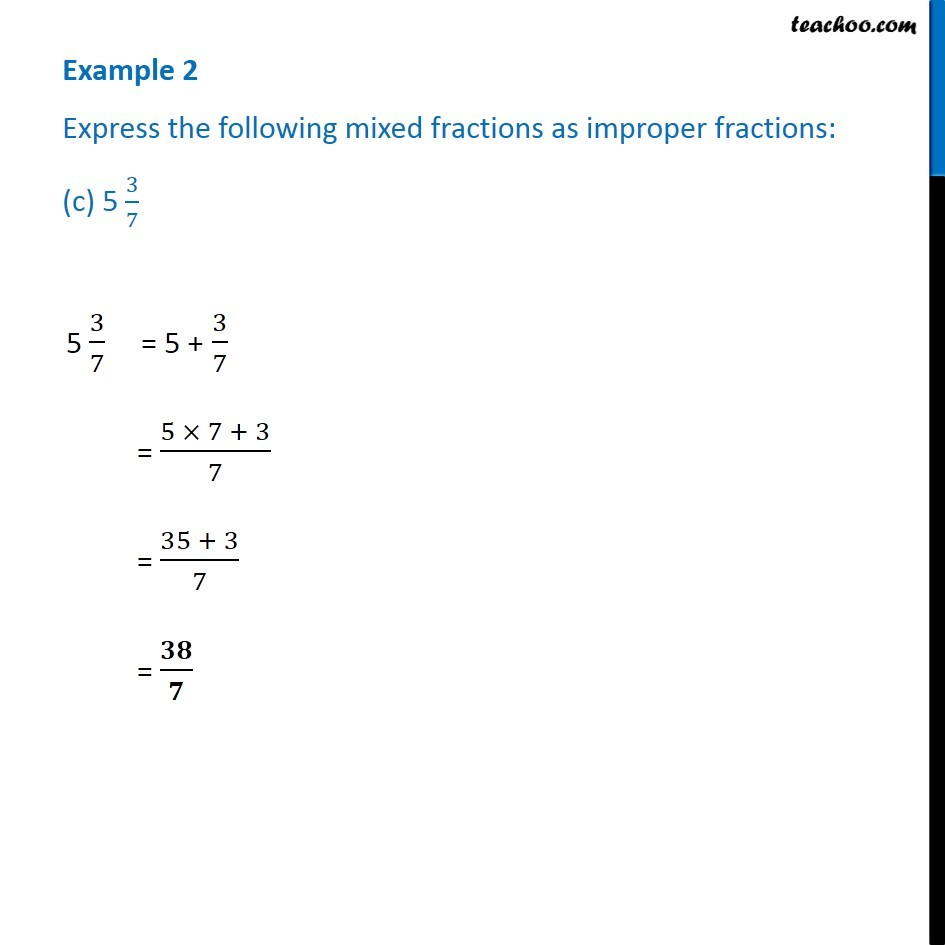Subscribe to our Youtube Channel - https://you.tube/teachoo

1. Chapter 7 Class 6 Fractions
2. Concept wise
3. Proper, improper and mixed fraction

Transcript

Example 2 Express the following mixed fractions as improper fractions: (a) 2 3/4 2 3/4 = 2 + 3/4 = (2 × 4 + 3)/4 = (8 + 3)/4 = 𝟏𝟏/𝟒 Example 2 Express the following mixed fractions as improper fractions: (b) 7 1/9 7 1/9 = 7 + 1/9 = (7 × 9 + 1)/9 = (63 + 1)/9 = 𝟔𝟒/𝟗 Example 2 Express the following mixed fractions as improper fractions: (c) 5 3/7 5 3/7 = 5 + 3/7 = (5 × 7 + 3)/7 = (35 + 3)/7 = 𝟑𝟖/𝟕

Proper, improper and mixed fraction